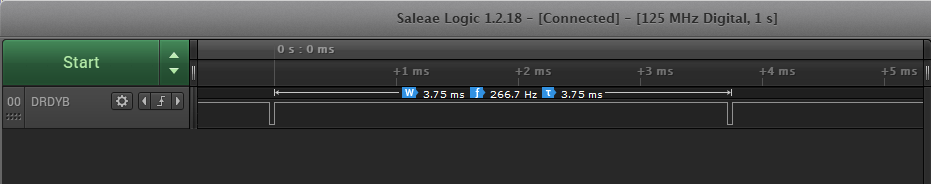If you have a related question, please click the "Ask a related question" button in the top right corner. The newly created question will be automatically linked to this question.

# ADS1293: Sampling rate differs from datasheet

Hi!

We're using the ADS1293 and have it set up to do output data at 267HZ but the DRDY pin is just toggling at 260Hz. Is there any reason for this/is this expected behavior?

Please let me verify this with the EVM and get back to you. Is it possible to share the complete device register setting?

Thanks

-TC

I have tested a few digital filters setting to get the ODR of 267Hz with an internal oscillator. I was able to observe the ODR of 267Hz on my logic analyzer. Please see the plots below for reference.

SDM Running at 102.4kHz.

R1=4, R2=6, R3=16R1=4, R2=8, R3=12R1=2, R2=6, R3=32Equation 5 in the datasheet shows ODR is derived from the clock frequency of the modulator and the digital filter setup (R1, R2, and R3). I would verify the internal clock frequency to the device is correct by probing the device's CLK pin.

Thanks

-TC

• Thanks! The internal clock is at 400kHz, is that the correct value?